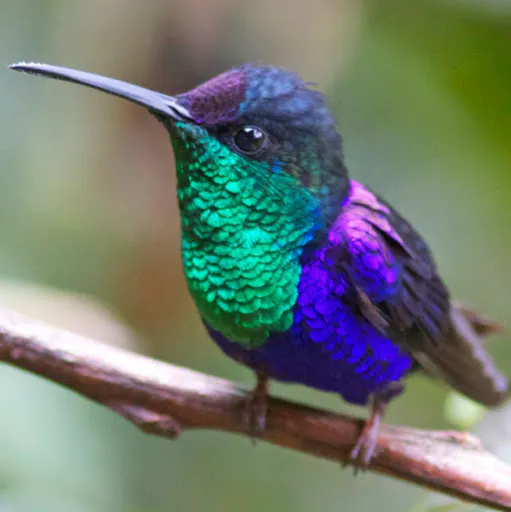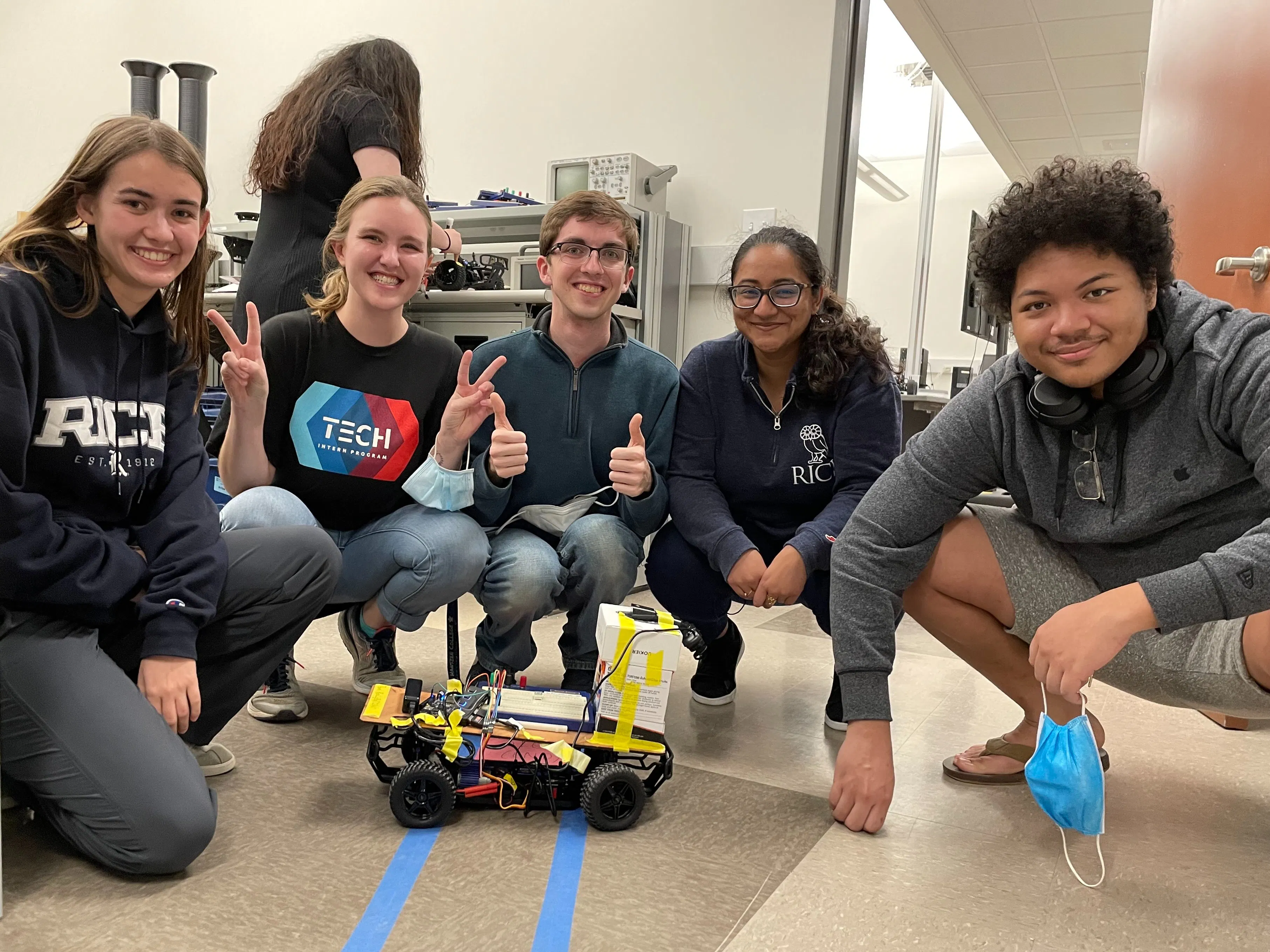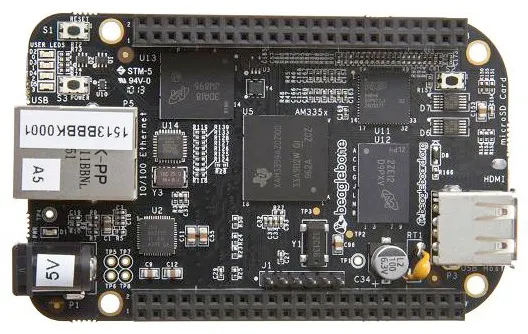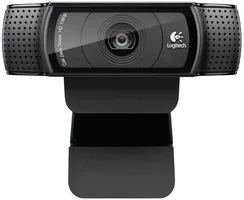# Autonomous path following car

This car follows a path on the ground, stops at a stop sign, and a stoplight due to the magic of vision processing on a BeagleBone Black!

IntermediateShowcase (no instructions)6 hours751## Things used in this project

### Hardware componentsBeagleBoard.org BeagleBone Black
×1Webcam, Logitech® HD Pro
×1
×1
 4 to 1 USB Hub - Sabrent
×1

### Software apps and online servicesOpenCV

## Code

### LaneKeepingAlgorithm.py

Python
Main code necessary for running the RC car with lane detection
```import cv2
import numpy as np
import matplotlib.pyplot as plt
import math
import sys
import time

# based on: https://www.instructables.com/Autonomous-Lane-Keeping-Car-Using-Raspberry-Pi-and/

# Throttle
throttlePin = "P8_13"
go_forward = 7.91
go_faster_tick_delay = 80
go_faster_tick = 0  # Do not change this here. Code will set this value after seeing stop sign
dont_move = 7.5

# Steering
steeringPin = "P9_14"
left = 9
right = 6

# Max number of loops
max_ticks = 2000

# Booleans for handling stop light
passedStopLight = False
atStopLight = False
passedFirstStopSign = False

secondStopLightTick = 0

def getRedFloorBoundaries():
"""
Gets the hsv boundaries and success boundaries indicating if the floor is red
:return: [[lower color and success boundaries for red floor], [upper color and success boundaries for red floor]]
"""
return getBoundaries("redboundaries.txt")

def isRedFloorVisible(frame):
"""
Detects whether or not the floor is red
:param frame: Image
:return: [(True is the camera sees a red on the floor, false otherwise), video output]
"""
print("Checking for floor stop")
boundaries = getRedFloorBoundaries()
return isMostlyColor(frame, boundaries)

def getTrafficRedLightBoundaries():
"""
Gets the traffic red light hsv boundaries and success boundaries
:return: [[lower color and success boundaries for red light], [upper color and success boundaries for red light]]
"""
return getBoundaries("trafficRedBoundaries.txt")

def isTrafficRedLightVisible(frame):
"""
Detects whether or not we can see a stop sign
:param frame:
:return: [(True is the camera sees a stop light, false otherwise), video output]
"""
print("Checking for traffic stop")
boundaries = getTrafficRedLightBoundaries()
return isMostlyColor(frame, boundaries)

def getTrafficGreenLightBoundaries():
"""
Gets the traffic green light hsv boundaries and success boundaries
:return: [[lower color and success boundaries for green light], [upper color and success boundaries for green light]]
"""
return getBoundaries("trafficGreenboundaries.txt")

def isTrafficGreenLightVisible(frame):
"""
Detects whether or not we can see a green traffic light
:param frame:
:return: [(True is the camera sees a green light, false otherwise), video output]
"""
print("Checking For Green Light")
boundaries = getTrafficGreenLightBoundaries()
return isMostlyColor(frame, boundaries)

def isMostlyColor(image, boundaries):
"""
Detects whether or not the majority of a color on the screen is a particular color
:param image:
:param boundaries: [[color boundaries], [success boundaries]]
:return: boolean if image satisfies provided boundaries, and an image used for debugging
"""
#Convert to HSV color space
hsv_img = cv2.cvtColor(image, cv2.COLOR_BGR2HSV)

#parse out the color boundaries and the success boundaries
color_boundaries = boundaries
percentage = boundaries

lower = np.array(color_boundaries)
upper = np.array(color_boundaries)

#Calculate what percentage of image falls between color boundaries
print("percentage_detected " + str(percentage_detected) + " lower " + str(lower) + " upper " + str(upper))
# If the percentage percentage_detected is betweeen the success boundaries, we return true, otherwise false for result
result = percentage < percentage_detected <= percentage
if result:
print(percentage_detected)
return result, output

def getBoundaries(filename):
"""
Reads the boundaries from the file filename
Format:
 lower: [H, S, V, lower percentage for classification of success]
 upper: [H, S, V, upper percentage for classification of success]
:param filename: file containing boundary information as above
:return: [[lower color and success boundaries], [upper color and success boundaries]]
"""
default_lower_percent = 50
default_upper_percent = 100
with open(filename, "r") as f:
lower_data = [val for val in boundaries.split(",")]
upper_data = [val for val in boundaries.split(",")]

if len(lower_data) >= 4:
lower_percent = float(lower_data)
else:
lower_percent = default_lower_percent

if len(upper_data) >= 4:
upper_percent = float(upper_data)
else:
upper_percent = default_upper_percent

lower = [int(x) for x in lower_data[:3]]
upper = [int(x) for x in upper_data[:3]]
boundaries = [lower, upper]
percentages = [lower_percent, upper_percent]
return boundaries, percentages

def initialize_car():
# give 7.5% duty at 50Hz to throttle
PWM.start(throttlePin, dont_move, frequency=50)

# wait for car to be ready
input()
PWM.start(steeringPin, dont_move, frequency=50)

def stop():
"""
Stops the car
:return: none
"""
PWM.set_duty_cycle(throttlePin, dont_move)

def go():
"""
Sends the car forward at a default PWM
:return: none
"""
PWM.set_duty_cycle(throttlePin, go_forward)

def go_faster():
"""
Sends the car forward at a faster default PWM
:return: none
"""

def go_backwards():
"""
(Attempts to) send the car backwards
:return: none
"""
PWM.set_duty_cycle(throttlePin, 7.1)

def detect_edges(frame):
# filter for blue lane lines
hsv = cv2.cvtColor(frame, cv2.COLOR_BGR2HSV)
# cv2.imshow("HSV",hsv)
lower_blue = np.array([90, 120, 0], dtype="uint8")
upper_blue = np.array([150, 255, 255], dtype="uint8")

# detect edges
# cv2.imshow("edges",edges)

return edges

def region_of_interest(edges):
height, width = edges.shape

# only focus lower half of the screen
polygon = np.array([[
(0, height),
(0, height / 2),
(width, height / 2),
(width, height),
]], np.int32)

# cv2.imshow("roi",cropped_edges)

return cropped_edges

def detect_line_segments(cropped_edges):
rho = 1
theta = np.pi / 180
min_threshold = 10

line_segments = cv2.HoughLinesP(cropped_edges, rho, theta, min_threshold,
np.array([]), minLineLength=5, maxLineGap=150)

return line_segments

def average_slope_intercept(frame, line_segments):
lane_lines = []

if line_segments is None:
print("no line segments detected")
return lane_lines

height, width, _ = frame.shape
left_fit = []
right_fit = []

boundary = 1 / 3
left_region_boundary = width * (1 - boundary)
right_region_boundary = width * boundary

for line_segment in line_segments:
for x1, y1, x2, y2 in line_segment:
if x1 == x2:
print("skipping vertical lines (slope = infinity")
continue

fit = np.polyfit((x1, x2), (y1, y2), 1)
slope = (y2 - y1) / (x2 - x1)
intercept = y1 - (slope * x1)

if slope < 0:
if x1 < left_region_boundary and x2 < left_region_boundary:
left_fit.append((slope, intercept))
else:
if x1 > right_region_boundary and x2 > right_region_boundary:
right_fit.append((slope, intercept))

left_fit_average = np.average(left_fit, axis=0)
if len(left_fit) > 0:
lane_lines.append(make_points(frame, left_fit_average))

right_fit_average = np.average(right_fit, axis=0)
if len(right_fit) > 0:
lane_lines.append(make_points(frame, right_fit_average))

return lane_lines

def make_points(frame, line):
height, width, _ = frame.shape

slope, intercept = line

y1 = height  # bottom of the frame
y2 = int(y1 / 2)  # make points from middle of the frame down

if slope == 0:
slope = 0.1

x1 = int((y1 - intercept) / slope)
x2 = int((y2 - intercept) / slope)

return [[x1, y1, x2, y2]]

def display_lines(frame, lines, line_color=(0, 255, 0), line_width=6):
line_image = np.zeros_like(frame)

if lines is not None:
for line in lines:
for x1, y1, x2, y2 in line:
cv2.line(line_image, (x1, y1), (x2, y2), line_color, line_width)

line_image = cv2.addWeighted(frame, 0.8, line_image, 1, 1)

return line_image

def display_heading_line(frame, steering_angle, line_color=(0, 0, 255), line_width=5):
height, width, _ = frame.shape

steering_angle_radian = steering_angle / 180.0 * math.pi

x1 = int(width / 2)
y1 = height
x2 = int(x1 - height / 2 / math.tan(steering_angle_radian))
y2 = int(height / 2)

cv2.line(heading_image, (x1, y1), (x2, y2), line_color, line_width)

def get_steering_angle(frame, lane_lines):
height, width, _ = frame.shape

if len(lane_lines) == 2:
_, _, left_x2, _ = lane_lines
_, _, right_x2, _ = lane_lines
mid = int(width / 2)
x_offset = (left_x2 + right_x2) / 2 - mid
y_offset = int(height / 2)

elif len(lane_lines) == 1:
x1, _, x2, _ = lane_lines
x_offset = x2 - x1
y_offset = int(height / 2)

elif len(lane_lines) == 0:
x_offset = 0
y_offset = int(height / 2)

angle_to_mid_deg = int(angle_to_mid_radian * 180.0 / math.pi)
steering_angle = angle_to_mid_deg + 90

return steering_angle

def plot_pd(p_vals, d_vals, error, show_img=False):
fig, ax1 = plt.subplots()
t_ax = np.arange(len(p_vals))
ax1.plot(t_ax, p_vals, '-', label="P values")
ax1.plot(t_ax, d_vals, '-', label="D values")
ax2 = ax1.twinx()
ax2.plot(t_ax, error, '--r', label="Error")

ax1.set_xlabel("Frames")
ax1.set_ylabel("PD Value")
ax2.set_ylim(-90, 90)
ax2.set_ylabel("Error Value")

plt.title("PD Values over time")
fig.legend()
fig.tight_layout()
plt.savefig("pd_plot.png")

if show_img:
plt.show()
plt.clf()

def plot_pwm(speed_pwms, turn_pwms, error, show_img=False):
fig, ax1 = plt.subplots()
t_ax = np.arange(len(speed_pwms))
ax1.plot(t_ax, speed_pwms, '-', label="Speed PWM")
ax1.plot(t_ax, turn_pwms, '-', label="Steering PWM")
ax2 = ax1.twinx()
ax2.plot(t_ax, error, '--r', label="Error")

ax1.set_xlabel("Frames")
ax1.set_ylabel("PWM Values")
ax2.set_ylabel("Error Value")

plt.title("PWM Values over time")
fig.legend()
plt.savefig("pwm_plot.png")

if show_img:
plt.show()
plt.clf()

# set up the car throttle and steering PWMs
initialize_car()

# set up video
video = cv2.VideoCapture(0)
video.set(cv2.CAP_PROP_FRAME_WIDTH, 320)
video.set(cv2.CAP_PROP_FRAME_HEIGHT, 240)

# wait for video to load
time.sleep(1)

# PD variables
kp = 0.085
kd = kp * 0.1
lastTime = 0
lastError = 0

# counter for number of ticks
counter = 0

# start the engines
go()

# arrays for making the final graphs
p_vals = []
d_vals = []
err_vals = []
speed_pwm = []
steer_pwm = []
current_speed = go_forward

stopSignCheck = 1
sightDebug = False
isStopSignBool = False
while counter < max_ticks:
frame = cv2.resize(original_frame, (160, 120))
if sightDebug:
cv2.imshow("Resized Frame", frame)

# check for stop sign/traffic light every couple ticks
if ((counter + 1) % stopSignCheck) == 0:
# check for stop light
if not passedStopLight and not atStopLight:
trafficStopBool, _ = isTrafficRedLightVisible(frame)
print(trafficStopBool)
if trafficStopBool:
print("detected red light, stopping")
stop()
atStopLight = True
continue
# check for the first stop sign
elif passedStopLight and not passedFirstStopSign:
isStopSignBool, floorSight = isRedFloorVisible(frame)
if sightDebug:
cv2.imshow("floorSight", floorSight)
if isStopSignBool:
print("detected first stop sign, stopping")
stop()
time.sleep(2)
passedFirstStopSign = True
# this is used to not check for the second stop sign until many frames later
secondStopSignTick = counter + 200
# now check for stop sign less frequently
stopSignCheck = 3
# add a delay to calling go faster
go_faster_tick = counter + go_faster_tick_delay
print("first stop finished!")
# check for the second stop sign
elif passedStopLight and passedFirstStopSign and counter > secondStopSignTick:
isStop2SignBool, _ = isRedFloorVisible(frame)
print("is a floor stop: ", isStopSignBool)
if isStop2SignBool:
# last stop sign detected, exits while loop
print("detected second stop sign, stopping")
stop()
break

# makes car go faster, helps it have enough speed to get to the end of the course
if isStopSignBool and counter == go_faster_tick:
print("Going FASTER")
go_faster()

# look for green stop light while waiting after red stop light
if not passedStopLight and atStopLight:
print("waiting at red light")
trafficGoBool, _ = isTrafficGreenLightVisible(frame)
if trafficGoBool:
passedStopLight = True
atStopLight = False
print("green light!")
go()
else:
continue

# process the frame to determine the desired steering angle
# cv2.imshow("original",frame)
edges = detect_edges(frame)
roi = region_of_interest(edges)
line_segments = detect_line_segments(roi)
lane_lines = average_slope_intercept(frame, line_segments)
lane_lines_image = display_lines(frame, lane_lines)
steering_angle = get_steering_angle(frame, lane_lines)

# calculate changes for PD
now = time.time()
dt = now - lastTime
if sightDebug:
cv2.imshow("Cropped sight", roi)
deviation = steering_angle - 90

# PD Code
error = -deviation
base_turn = 7.5
proportional = kp * error
derivative = kd * (error - lastError) / dt

# take values for graphs
p_vals.append(proportional)
d_vals.append(derivative)
err_vals.append(error)

# determine actual turn to do
turn_amt = base_turn + proportional + derivative

# caps turns to make PWM values
if 7.2 < turn_amt < 7.8:
turn_amt = 7.5
elif turn_amt > left:
turn_amt = left
elif turn_amt < right:
turn_amt = right

# turn!
PWM.set_duty_cycle(steeringPin, turn_amt)

# take values for graphs
steer_pwm.append(turn_amt)
speed_pwm.append(current_speed)

# update PD values for next loop
lastError = error
lastTime = time.time()

key = cv2.waitKey(1)
if key == 27:
break

counter += 1

# clean up resources
video.release()
cv2.destroyAllWindows()
PWM.set_duty_cycle(throttlePin, 7.5)
PWM.set_duty_cycle(steeringPin, 7.5)
PWM.stop(throttlePin)
PWM.stop(steeringPin)
PWM.cleanup()

plot_pd(p_vals, d_vals, err_vals, True)
plot_pwm(speed_pwm, steer_pwm, err_vals, True)
```

### trafficRedBoundaries.txt

Plain text
Color and success boundaries for computer vision to recognize traffic red light
```140, 20, 100, 0.6
179, 80, 255
```

### trafficGreenboundaries.txt

Plain text
Color and success boundaries for computer vision to recognize traffic green light
```40 , 50, 84, 1
90, 255, 255, 100
```

### redboundaries.txt

Plain text
Color and success boundaries for computer vision to recognize red stop sign on floor
```140 , 90, 200, 0.003
200, 255, 250
```

## Credits

### Daniel Rothfusz

1 project • 4 followers

### Lauren Ivory

1 project • 2 followers

### Rashi Bose

1 project • 2 followers

### Isabelle Scott

1 project • 3 followers

### Brandon Stanley

1 project • 2 followers
Thanks to raja_961.